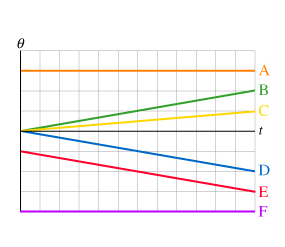# Problem: The figure shows angular position versus time graphs for six different objects.Rank these graphs on the basis of the angular acceleration of the object. Rank positive angular accelerations as larger than negative angular accelerations.Rank from largest to smallest. To rank items as equivalent, overlap them.

###### FREE Expert Solution

Angular acceleration:

$\overline{){\mathbf{\alpha }}{\mathbf{=}}\frac{\mathbf{d}\mathbf{\omega }}{\mathbf{d}\mathbf{t}}}$

Graphs A and F are not changing the angular position.

This means that the change in the angular velocity is zero.

Therefore, α = 0/dt = 0 rad/s2

84% (465 ratings)###### Problem Details

The figure shows angular position versus time graphs for six different objects.Rank these graphs on the basis of the angular acceleration of the object. Rank positive angular accelerations as larger than negative angular accelerations.

Rank from largest to smallest. To rank items as equivalent, overlap them.